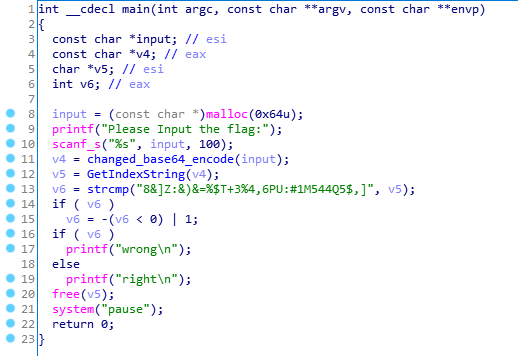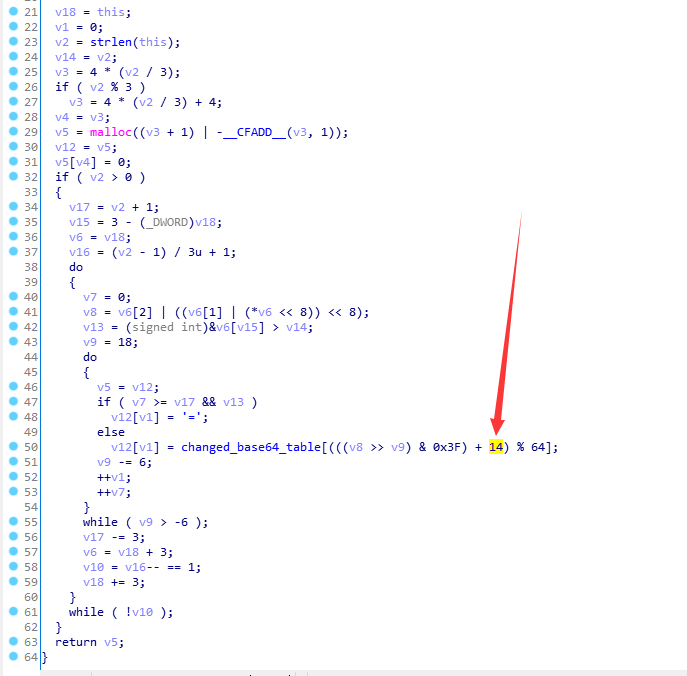# 0x00 前言

pwn没给libc，用libcsearcher也不管用，导致远程一直没打通，但是事后询问了一位师傅，他直接大胆猜测libc就是2.23的…也确实打通了…看来我还是缺少经验555

# 0x01 分析## changed_base64_encode## GetIndexString

`v5[v6 + 1] = ((second >> 4) + 32) | 16 * (first & 3);`

### 隐含的问题

#### 情况一

`((second >> 4) + 32)` == `100111`

`16 * (first & 3)` == `110000`

`((second >> 4) + 32) | 16 * (first & 3)` == `110111`

`((second >> 4))` == `000111`

`16 * (first & 3)` == `110000`

`((second >> 4)) | 16 * (first & 3)` == `110111`

#### 情况二

`((second >> 4) + 32)` == `100111`

`16 * (first & 3)` == `010000`

`((second >> 4) + 32) | 16 * (first & 3)` == `110111`

`((second >> 4))` == `000111`

`16 * (first & 3)` == `010000`

`((second >> 4)) | 16 * (first & 3)` == `010111` == `(((second >> 4) + 32) | 16 * (first & 3))-32`

# 0x04 写在最后-------------至此本文结束感谢您的阅读-------------